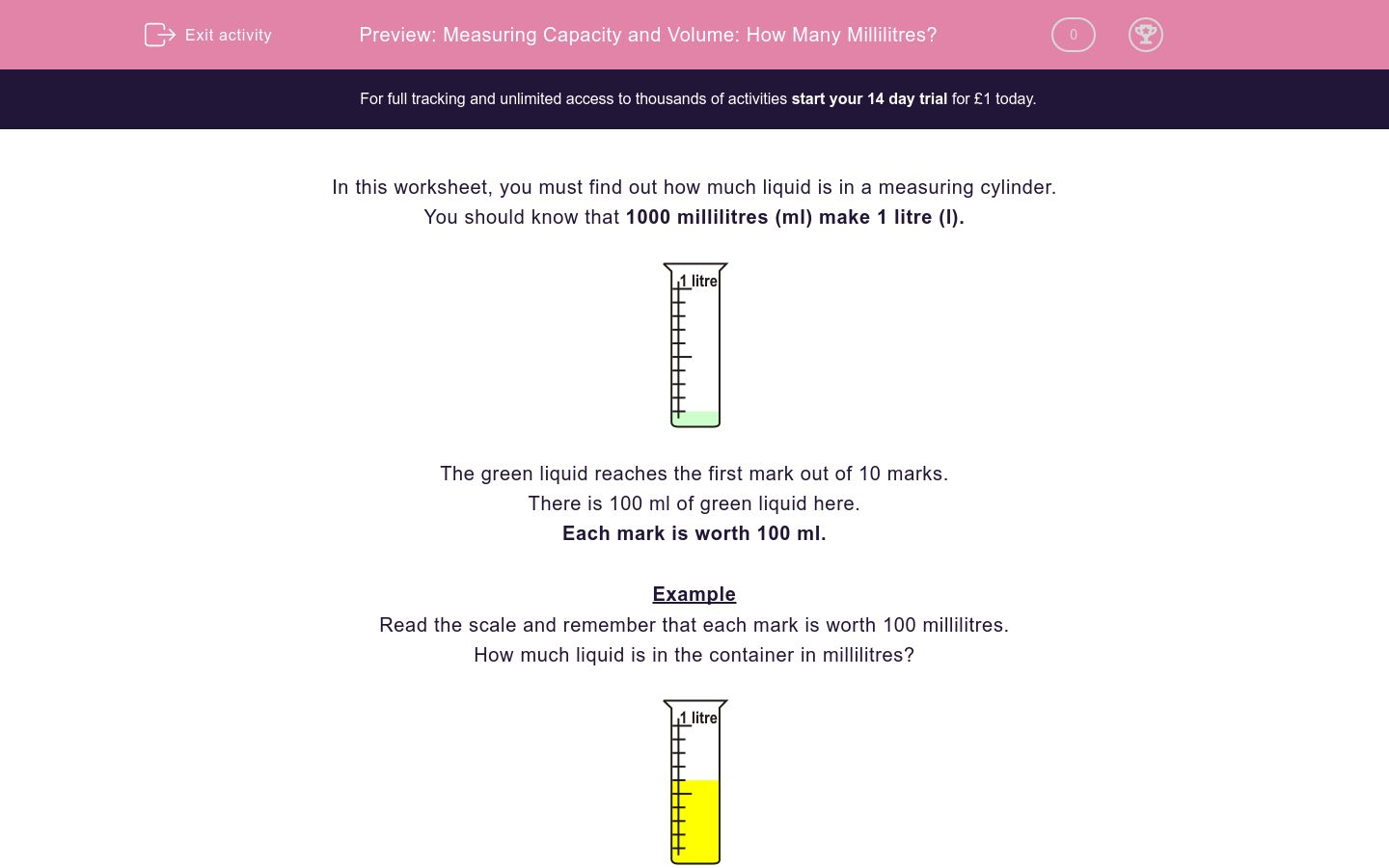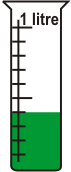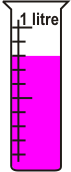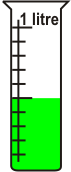# Measuring Capacity and Volume: How Many Millilitres?

In this worksheet, students find out how much liquid is in a measuring cylinder.Key stage:  KS 2

Curriculum topic:   Maths and Numerical Reasoning

Difficulty level:### QUESTION 1 of 10

In this worksheet, you must find out how much liquid is in a measuring cylinder.

You should know that 1000 millilitres (ml) make 1 litre (l).The green liquid reaches the first mark out of 10 marks.

There is 100 ml of green liquid here.

Each mark is worth 100 ml.

Example

Read the scale and remember that each mark is worth 100 millilitres.

How much liquid is in the container in millilitres?The liquid reaches the 6th mark.

600 ml

Read the scale and remember that each mark is 100 millilitres.

How much liquid is in the container in millilitres?

Just write the numberRead the scale and remember that each mark is 100 millilitres.

How much liquid is in the container in millilitres?

Just write the numberRead the scale and remember that each mark is 100 millilitres.

How much liquid is in the container in millilitres?

Just write the numberRead the scale and remember that each mark is 100 millilitres.

How much liquid is in the container in millilitres?

Just write the numberRead the scale and remember that each mark is 100 millilitres.

How much liquid is in the container in millilitres?

Just write the numberRead the scale and remember that each mark is 100 millilitres.

How much liquid is in the container in millilitres?

Just write the numberRead the scale and remember that each mark is 100 millilitres.

How much liquid is in the container in millilitres?

Just write the numberRead the scale and remember that each mark is 100 millilitres.

How much liquid is in the container in millilitres?

Just write the numberRead the scale and remember that each mark is 100 millilitres.

How much liquid is in the container in millilitres?

Just write the numberRead the scale and remember that each mark is 100 millilitres.

How much liquid is in the container in millilitres?

Just write the number• Question 1

Read the scale and remember that each mark is 100 millilitres.

How much liquid is in the container in millilitres?

Just write the number200
EDDIE SAYS
2nd mark.
• Question 2

Read the scale and remember that each mark is 100 millilitres.

How much liquid is in the container in millilitres?

Just write the number300
EDDIE SAYS
3rd mark.
• Question 3

Read the scale and remember that each mark is 100 millilitres.

How much liquid is in the container in millilitres?

Just write the number900
EDDIE SAYS
9th mark.
• Question 4

Read the scale and remember that each mark is 100 millilitres.

How much liquid is in the container in millilitres?

Just write the number400
EDDIE SAYS
4th mark.
• Question 5

Read the scale and remember that each mark is 100 millilitres.

How much liquid is in the container in millilitres?

Just write the number800
EDDIE SAYS
8th mark.
• Question 6

Read the scale and remember that each mark is 100 millilitres.

How much liquid is in the container in millilitres?

Just write the number500
EDDIE SAYS
5th mark.
• Question 7

Read the scale and remember that each mark is 100 millilitres.

How much liquid is in the container in millilitres?

Just write the number700
EDDIE SAYS
7th mark.
• Question 8

Read the scale and remember that each mark is 100 millilitres.

How much liquid is in the container in millilitres?

Just write the number100
EDDIE SAYS
1st mark.
• Question 9

Read the scale and remember that each mark is 100 millilitres.

How much liquid is in the container in millilitres?

Just write the number600
EDDIE SAYS
6th mark.
• Question 10

Read the scale and remember that each mark is 100 millilitres.

How much liquid is in the container in millilitres?

Just write the number1000
EDDIE SAYS
10th mark.
---- OR ----

Sign up for a £1 trial so you can track and measure your child's progress on this activity.

### What is EdPlace?

We're your National Curriculum aligned online education content provider helping each child succeed in English, maths and science from year 1 to GCSE. With an EdPlace account you’ll be able to track and measure progress, helping each child achieve their best. We build confidence and attainment by personalising each child’s learning at a level that suits them.

Get started